By jwyang
• Jan 1, 1010

# Jia Xian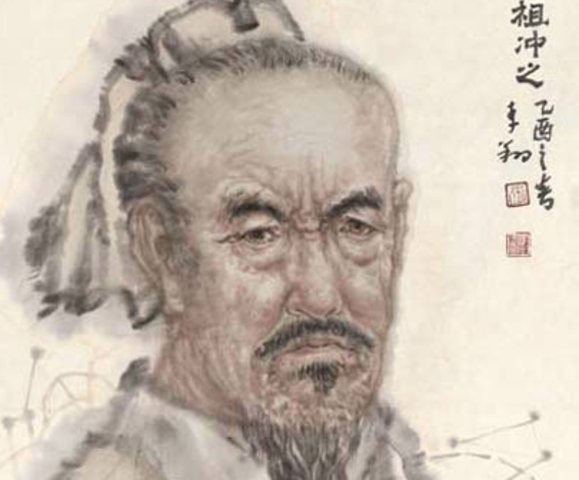• Jul 18, 1013

• Jan 1, 1031

# Shen Kuo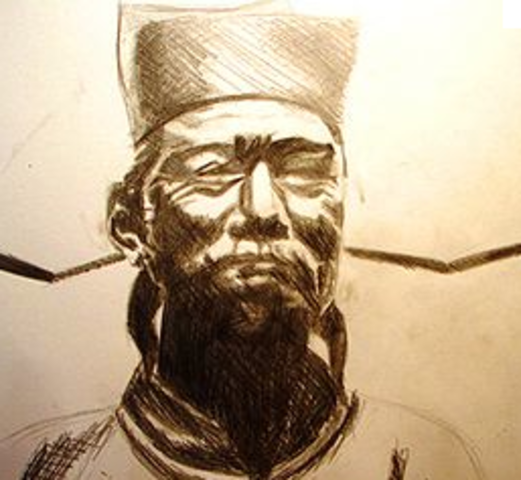• Jan 1, 1080• Jan 1, 1114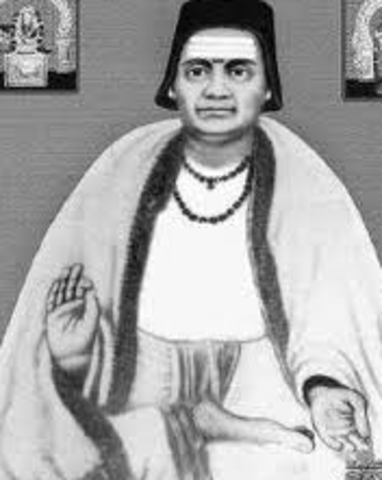• Jan 1, 1170

# Leonardo Fibonacci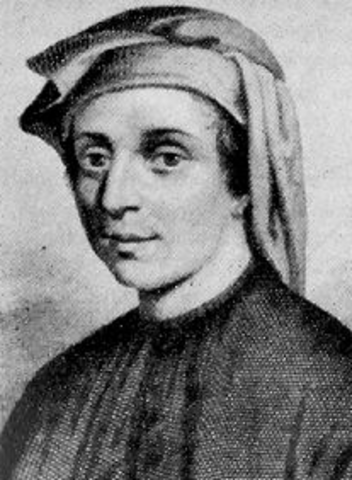• Jan 1, 1192

# Li Zhi• Apr 12, 1202

# Qin Jiushao• Jan 1, 1214

# Roger Bacon• Jan 1, 1215

# william of Moerbeke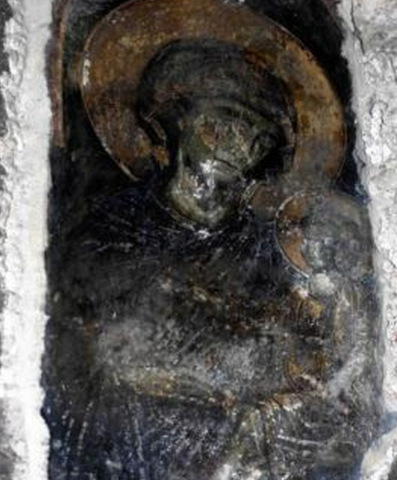• Jan 1, 1220

# Campanus of Novara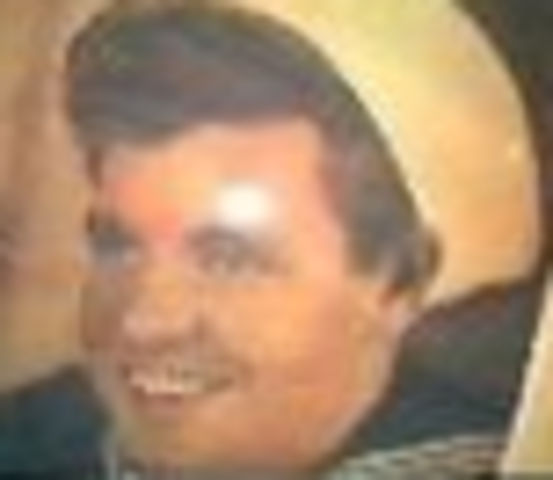• Jan 1, 1225

# Jordanus Nemorarius• Apr 13, 1230

# Witelo• Jan 1, 1238

# Yang Hui• Apr 13, 1271

# Guo Shouging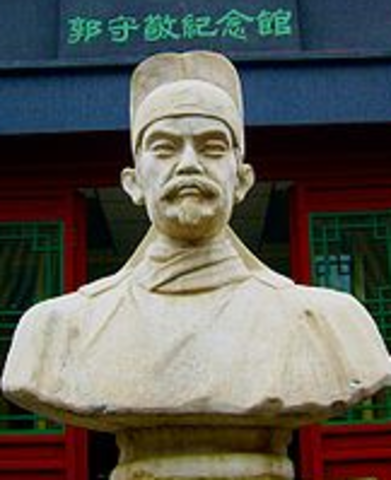• Jan 1, 1380

# Parameshvara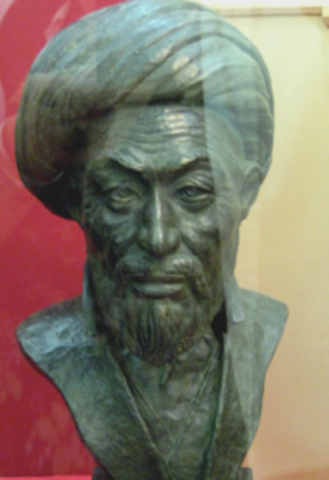• Feb 6, 1465

# Scipione del Ferro

Italian mathematician who first discovered a method to solve the depressed cubic equation.
• Jan 1, 1492

Adam Ries is generally considered to be the "father of modern calculating". He decisively contributed to the recognition that Roman numerals are unwieldy in practice and to their replacement by the considerably more structured Arabic numerals to a large extent
• Jan 1, 1500

# Niccolò Fontana Tartaglia

Tartaglia is perhaps best known today for his conflicts with Gerolamo Cardano. Cardano cajoled Tartaglia into revealing his solution to the cubic equations Known for solving cubic equations called Cardano-Tartaglia Formula
• Sep 24, 1501

# Gerolamo Cardano

His gambling led him to formulate elementary rules in probability, making him one of the founders of the field. Cardano was the first mathematician to make systematic use of numbers less than zero He solution to one particular case of the cubic equation 0= ax^3+bx^2+c (in modern notation)
• Jan 1, 1502

# Pedro Nunes

Nunes was considered to be one of the greatest mathematicians of his time. He is best known for his contributions in the technical field of navigation, He was the first to propose the idea of a loxodrome and was also the inventor of several measuring devices, including the nonius. Nunes also solved the problem of finding the day with the shortest twilight duration, for any given position, and its duration.
• Dec 9, 1508

# Gemma Frisius

He created important globes, improved the mathematical instruments of his day and applied mathematics in new ways to surveying and navigation.
• Jan 1, 1512

# Robert Recorde

He introduced the "equals" sign (=) in 1557.
• Feb 2, 1522

# Lodovico Ferrari

Ferrari aided Cardano on his solutions for quadratic equations and cubic equations, and was mainly responsible for the solution of quartic equations that Cardano published.
• Jan 1, 1550

# John Napier

Best known for:
- Logarithms
- Napier's bones
- Decimal notation
• Feb 15, 1564

# Galileo Galilei

Been called the:
"father of modern observational astronomy",
"father of modern physics",
"father of science",
"the Father of Modern Science". Known for:
Kinematics
Dynamics
Telescopic observational astronomy
Heliocentrism
• # René Descartes

Known for the Cartesian coordinate system and Cartesian dualism, He has been dubbed the 'Father of Modern Philosophy" Descartes was also one of the key figures in the Scientific Revolution. He is credited as the father of analytical geometry, the bridge between algebra and geometry, crucial to the discovery of infinitesimal calculus and analysis.
• # Pierre de Fermat

An amateur mathematician who is given credit for early developments that led to infinitesimal calculus, including his adequality. He is recognized for his discovery of an original method of finding the greatest and the smallest ordinates of curved lines. He made notable contributions to analytic geometry, probability, and optics. He is best known for Fermat's Last Theorem.
• # Blaise Pascal

He invented the mechanical calculator. Known for:
- Pascal's triangle
- Pascal's law
- Pascal's theorem
• # Isaac Newton

Has been "considered by many to be the greatest and most influential scientist who ever lived." Known for:
Newtonian mechanics
Universal gravitation
Infinitesimal calculus
Optics
Binomial series
Newton's method
Philosophiæ Naturalis Principia Mathematica
• # Jacob Bernoulli

Was one of the many prominent mathematicians in the Bernoulli family. Bernoulli discovered the constant e by studying a question about compound interest Known for:
Bernoulli differential equation
Bernoulli numbers
Bernoulli's inequality
• # Guillaume de l'Hôpital

His name is firmly associated with l'Hôpital's rule for calculating limits involving indeterminate forms 0/0 and ∞/∞. Known for:
Infinitesimal calculus
Differential geometry of curves
• # Brook Taylor

English mathematician who is best known for Taylor's theorem and the Taylor series
• # James Stirling

Best known for The Stirling numbers and Stirling's approximation
• # Leonhard Euler

He made important discoveries in fields as diverse as infinitesimal calculus and graph theory. He also introduced much of the modern mathematical terminology and notation, particularly for mathematical analysis, such as the notion of a mathematical function Contributed to:
Mathematical Notation
Analysis
Number Theory
Graph Theory
Applied Mathematics
Physics and Astronomy
Logic
• # Joseph Louis Lagrange

He made significant contributions to all fields of analysis, number theory, and classical and celestial mechanics. Known for Lagrange Theorem
• # Pierre-Simon Laplace

He formulated Laplace's equation, and pioneered the Laplace transform The Laplacian differential operator, widely used in mathematics, is also named after him.
• # Joseph Fourier

Best known for initiating the investigation of Fourier series and their applications to problems of heat transfer and vibrations. The Fourier transform and Fourier's Law are also named in his honour. Fourier is also generally credited with the discovery of the greenhouse effect.
• # Augustin-Louis Cauchy

French mathematician who was an early pioneer of analysis. Started the project of formulating and proving the theorems of infinitesimal calculus in a rigorous manner, rejecting the heuristic principle of the generality of algebra exploited He defined continuity in terms of infinitesimals and gave several important theorems in complex analysis and initiated the study of permutation groups in abstract algebra.
• # William Rowan Hamilton

Known for:
- Hamiltonian path
- Icosian Calculus
• # Augustus De Morgan

He formulated De Morgan's laws and introduced the term mathematical induction, making its idea rigorous
• # Pafnuty Chebyshev

Chebyshev is known for his work in the field of probability, statistics and number theory and The Bertrand–Chebyshev theorem
• # Arthur Cayley

He postulated the Cayley–Hamilton theorem He was the first to define the concept of a group in the modern way like permutation group Known for Cayley Table or Theorem
• # Oliver Heaviside

Invented mathematical techniques to the solution of differential equations (later found to be equivalent to Laplace transforms)